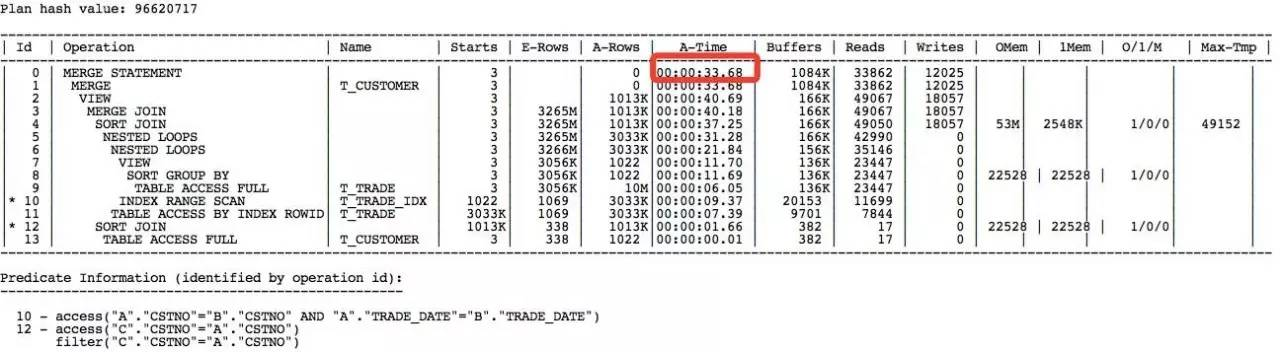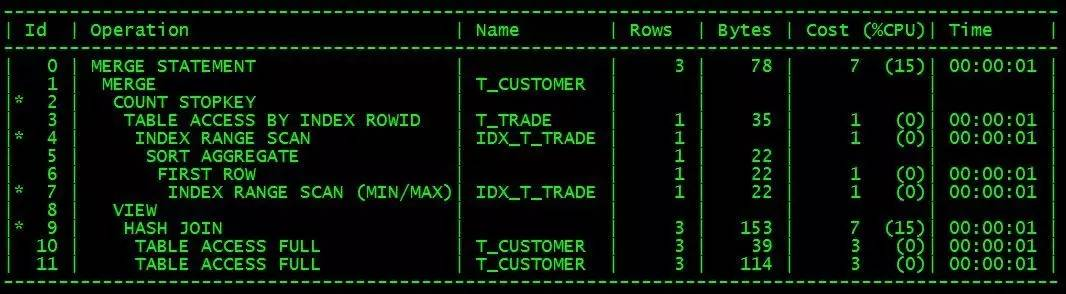# 数据分布决定SQL写法

merge into t_customer c using

(

select a.cstno, a.amount from t_trade a,

group by cstno) b

) m

on(c.cstno = m.cstno)

when matched then

update set c.amount = m.amount;merge into t_customer c using

(

select a.cstno,a.amount from

where RNO=1

)  m

on(c.cstno = m.cstno)

when matched then

update set c.amount = m.amount;

declare

vamount number;

begin

for v in (select * from t_customer )

loop

select amount into vamount from

where rownum<2;

update t_customer set amount = vamount where cstno=v.cstno;

end loop

commit;

end;

/

merge into t_customer c using

(

select tc.cstno,

(select amount

where td1.cstno=tc.cstno and td1.trade_date = (select max(trade_date) from t_trade td2 where tc.cstno = td2.cstno) and rownum=1 ) as amount

from t_customer tc

)  m

on(c.cstno = m.cstno)

when matched then

update set c.amount = m.amount;SQL优化，除了要避免低效的SQL写法，主要还是要看表的数据量与数据分布情况，plsql的改写方法，在少数比较特殊的情况下会体现出较高的效率，在某些数据分布的情况下，效率可能还不如原SQL。但是，优化思路非常值得借鉴。